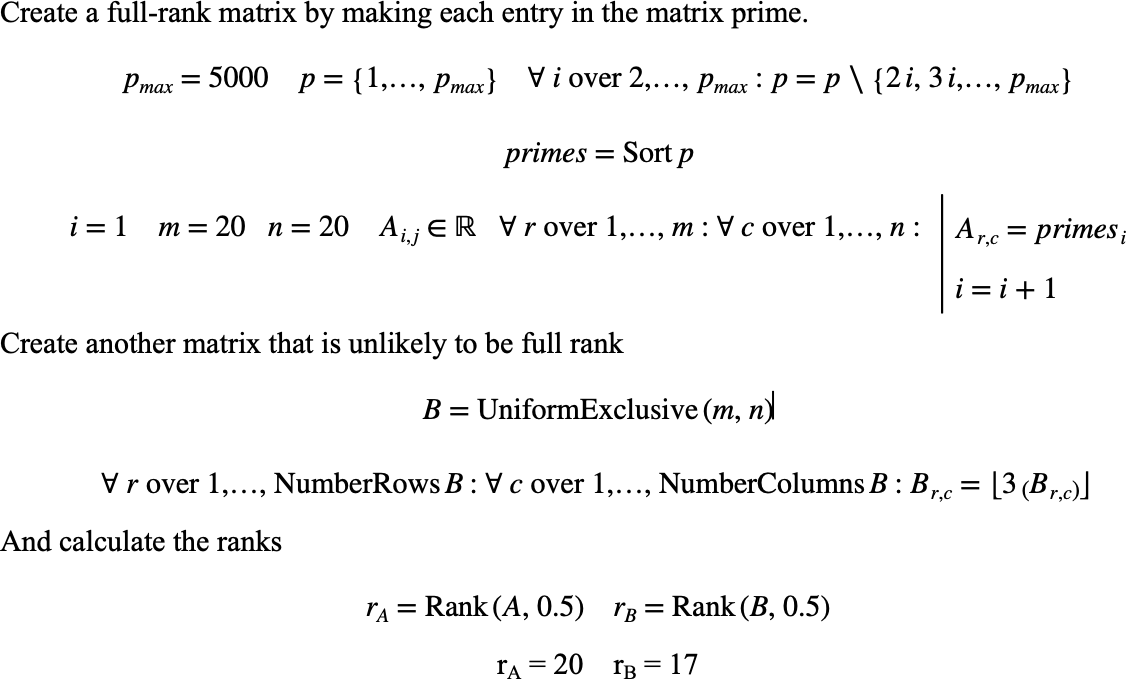# $$\text{Rank}$$¶

You can use the $$\text{Rank}$$ function to determine the rank of a matrix.

You can use the \rank backslash command to insert this function.

The following variants of this function are available:

• $$\text{integer } \text{Rank} \left ( \text{<matrix>}, \text{<tolerance>} \right )$$

The $$\text{<matrix>}$$ parameter is the matrix to determine the rank of. The $$\text{<tolerance>}$$ is a scalar tolerance to apply to the matrix rank calculation.

The $$\text{Rank}$$ function returns an integer value indicating the rank of the matrix.

The function calculates the matrix rank using singular value decomposition producing a square diagonal matrix $$\Sigma$$ and two semi-unitary matrices. The matrix rank is then determined by counting the number of diagonal entries in $$\Sigma$$ whose magnitude is greater than the provided tolerance.

Figure 188 shows the basic use of the $$\text{Rank}$$ function.Figure 188 Example Use Of The Rank Function